Function Repository Resource:

# PermutationCountByInversions

Get the number of permutations having a specified length and number of inversions

Contributed by: Wolfram Staff (original content by Sriram V. Pemmaraju and Steven S. Skiena)
 ResourceFunction["PermutationCountByInversions"][n,k] gives the number of permutations of length n with exactly k inversions. ResourceFunction["PermutationCountByInversions"][n] gives a List for all k starting at zero.

## Details and Options

ResourceFunction["PermutationCountByInversions"][n,k] is also the number of inversion vectors of n-permutations that sum up to k, or equivalently, the number of n-permutations with inversion count k.
ResourceFunction["PermutationCountByInversions"] effectively uses generating functions to find the number of permutations.
ResourceFunction["PermutationCountByInversions"][n,All] is equivalent to ResourceFunction["PermutationCountByInversions"][n].

## Examples

### Basic Examples (2)

The number of permutations of length 7 with 16 inversions:

 In:=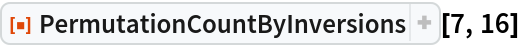Out=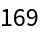The list of 7-permutations for all possible k:

 In:=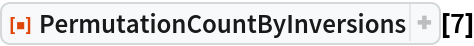Out=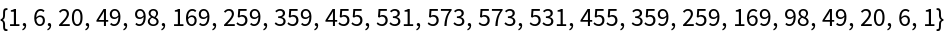### Scope (1)

Using All as the second argument is equivalent to the single-argument form:

 In:=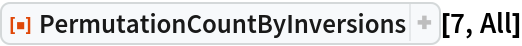Out=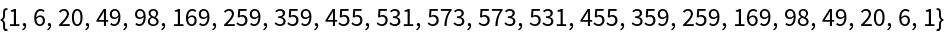In:=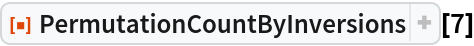Out=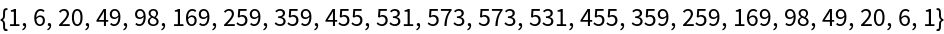### Properties and Relations (4)

Select 4-permutations with inversion count 3:

 In:=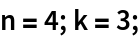In:=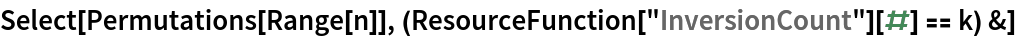Out=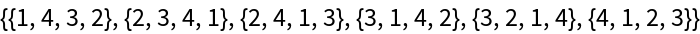The number of selected permutations is the permutation count by inversions:

 In:=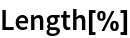Out=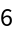In:=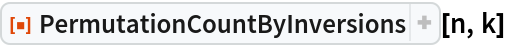Out=The list of permutation counts for all k is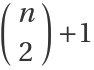long:

 In:=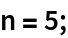In:=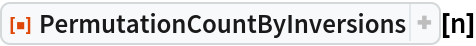Out=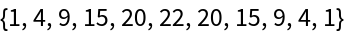In:=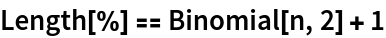Out=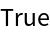There is always a single permutation with zero inversions:

 In:=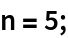In:=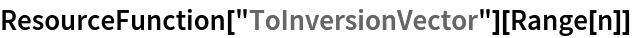Out=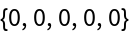In:=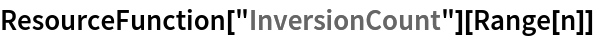Out=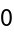In:=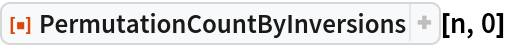Out=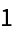The number of permutations with k inversions is equal to the number of permutations with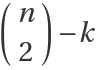inversions:

 In:=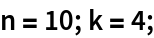In:=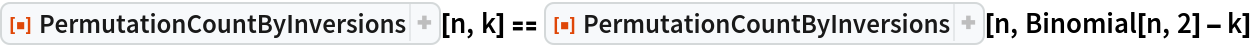Out=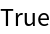In:=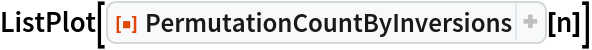Out=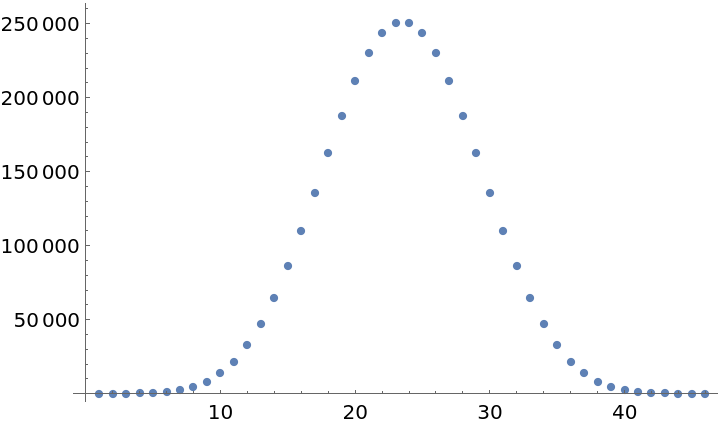## Version History

• 1.0.0 – 06 July 2020